## Introduction

Topological insulators have witnessed a lot of success in the last decade. In various electronic systems, from the artificially designed Haldane lattice1, to graphene2,3 and HgTe/CdTe quantum well structures4,5, the topology – a mathematical property describing the quantized behavior of the wavefunctions over the associated dispersion bands – has been found to have a profound influence on the transportation properties of electronic wave functions6,7. The concept of topology was thereafter borrowed from quantum systems and transplanted into classical systems, offering researchers a new degree of freedom in controlling and manipulating electromagnetic8,9,10,11,12,13,14,15,16,17,18,19,20,21,22,23,24,25,26,27, acoustic28,29,30,31,32,33 and elastic waves34,35,36,37,38,39,40,41,42 in their corresponding artificial structures.

Both electromagnetic and elastic waves satisfy vector wave equations. Electromagnetic wave has two transverse polarizations/modes that are perpendicular to the propagation direction. Elastic wave contains not only two transverse polarizations/modes but also one longitudinal polarization/mode. The polarization degree of freedom is naturally exploited to emulate spins in quantum systems in the realization of unidirectional propagation of classical waves. In contrast, acoustic wave satisfies scalar wave equation and has only one longitudinal polarization, making the realization of unidirectional propagation in acoustics nontrivial. Thus, the reported topological nontrivial phases in acoustic systems were enabled either by rotating fluids to break the time-reversal symmetry29,30,31, or by utilizing chiral interlayer coupling to break the inversion symmetry32. Although topologically protected edge states were numerically demonstrated in these works, the need of integrating rotational fluids into resonators or the fabrication of complex inversion-breaking chiral structures remains technically challenging. Very recently, another artificial structure was designed to realize the unidirectional propagation of acoustic waves33. The graphite-like structure is a bit complex, which involves two scatterers in each primitive unit cell, and its lattice constant has to be on the wavelength scale to support the associated unidirectional propagation. Here, we propose a different and simple way to realize topologically protected edge states for acoustic waves in the subwavelength region without introducing rotational fluids or complex unit cells.

The system is a two-dimensional acoustic crystal (AC) composed of a triangular array of core-shell cylinders embedded in a water host. We show that by utilizing the rotational symmetry of the unit cell, pseudo-time-reversal symmetry23 can be constructed and, as a result, the acoustic analogue of the quantum spin Hall effect can be emulated. We develop an effective Hamiltonian for the associated system, and unveil the inherent link between the band inversion and the topological phase transition. We unambiguously demonstrate topologically protected acoustic one-way edge states with robust propagation against scattering from defects.

## Results

### Design and characteristics

A schematic of the two-dimensional (2D) AC is shown in Fig. 1(a). Each core-shell cylinder has a steel rod with radius r as its core, which is coated by a layer of silicone rubber. The outer radius of the cylinder is R, and a is the lattice constant. The acoustic wave equation is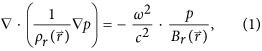where p is the pressure, with ρr = ρ/ρ0 and Br = B/B0 being the relative mass density and bulk modulus, respectively.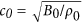is the speed of sound in water. The mass densities for water, rubber and steel are ρ0 = 1000kg/m3, ρ1 = 1300kg/m3, and ρ2 = 7670kg/m3, respectively. The longitudinal wave velocities in water, rubber and steel are c0 = 1490m/s, c1 = 489.9m/s, and c2 = 6010m/s, respectively. Due to the strong mismatch between the longitudinal velocities in these media, the shear wave modes in the solid components are ignored here, and this simplification does not alter the essential physics of the system43,44.

It was shown in ref. 44 that a four-fold degeneracy is achieved at the Г point at frequency ωD = 0.6092(2πc0/a) when r = 0.2822a and R = 0.3497a, and, as a result, a double Dirac cone was formed at the center of the Brillouin zone as shown in Fig. 1(b). Here, we use COMSOL Multiphysics, a commercial package based on the finite-element method, to calculate the band structures.

The four-fold degeneracy is realized when the doubly degenerate dipolar states coincide with the doubly degenerate quadrupolar states at ωD. The degeneracy is thus accidental. That is to say, if we alter the geometric parameters, e.g., the inner and/or outer radii of the core-shell cylinders, the four-fold degeneracy will be lifted, and the dipolar states will be separated from the quadrupolar states. In Fig. 1(c,d), we plot the band structures for r = 0.265a and R = 0.322a, and for r = 0.295a and R = 0.35a, respectively, where the four-fold degeneracy is lifted and the dipolar states are separated from the quadrupolar states.

For the AC we mentioned above, the point group at the center of the Brillouin zone (BZ) is C6v, which has two 2D irreducible representations45: E1 with basis functions (x,y) and E2 with basis functions (2xy, x2 − y2). E1 modes have odd spatial parity, while E2 modes have even spatial parity. From the distribution of the eigenfields shown in Fig. 2, it is easy to recognize that the E1 [Fig. 2(a,b)] and E2 [Fig. 2(c,d)] representations have, respectively, the same symmetry as the (px,py) and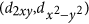orbitals of electrons in quantum systems. We note that relative eigenfrequencies corresponding to different irreducible representations change as the geometry of the core-shell cylinder changes. To be more specific, the eigenfrequency associated with the E1 representation is lower than that of E2 as shown in Fig. 1(c), but it is higher in Fig. 1(d), indicating that a band inversion process occurs as the geometry changes. Later, we will show that this band inversion is inherently associated with a topological phase transition.

In the Method section, we demonstrate that the spatial symmetry of the E1 and E2 representations can be utilized to construct pseudo-time-reversal symmetry. Here we refer only to the main results. From the E1 basis functions (px,py), we can construct the pseudo-spin states as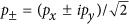, with p+ (p) being the pseudo spin-up (spin-down) state. The same conclusion can be made on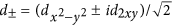, which is another pair of pseudo spin-up/spin-down states associated with the E2 representation.

### Effective Hamiltonian

To understand the topological property of the band gaps shown in Fig. 1(b,c), we construct an effective Hamiltonian for the current system around the Γ point from a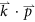perturbation method44,45,46. We assume Γα (α = 1, 2, 3, 4) are the four eigenstates at the Γ point: Γ1 = px, Γ2 = py,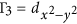, and Γ4 = d2xy, with the px(y) and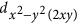states corresponding to the E1 and E2 representations, respectively. The effective Hamiltonian around the Γ point then is given by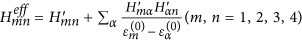, where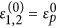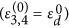is the eigenfrequency of Γ1,23,4), and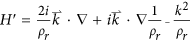is theperturbation term for the acoustic wave equation (1), obtained by expanding the Bloch eigenstates at point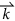as the linear combinations of the Bloch eigenstates at point Γ. Rewriting the above Hamiltonian on the basis [p+, d+, p, d], we arrive at the following effective Hamiltonian in the vicinity of the Γ point,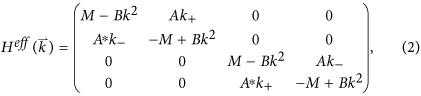wherek± = kx ± iky and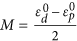is the frequency difference between E2 and E1 representations at the Γ point, which is positive (negative) before (after) the band inversion. A comes from off-diagonal elements of the first-order perturbation term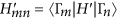with m = 1, 2 and n = 3, 4. B is determined by the diagonal elements of the second-order perturbation term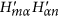, and is typically negative. We note that to derive the above Hamiltonian, the spatial symmetries of the eigenstates, Γα, are utilized. The effective Hamiltonian,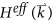, shown in Eq. (2) takes a similar form as that proposed in the Bernevig-Hughes-Zhang (BHZ) model for the CdTe/HgTe/CdTe quantum well system4.

We note that, in Fig. 1(c), the bands above (below) the gap belong to the E2 (E1) representation, which means that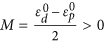. For the Hamiltonian expressed in Eq. (2), the spin Chern numbers can be evaluated as4,47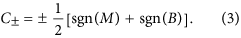Since C± = 0, we conclude that the band gap shown in Fig. 1(c) is trivial. However the situation is different in Fig. 1(d), where the bands above (below) the gap exhibit E1 (E2) characteristics around the Γ point, meaning that M < 0. Applying Eq. (3), we immediately know that C± = ±1 and the gap in Fig. 1(d) is nontrivial. It is evident that the topological property of the effective Hamiltonian is determined by the signs of M and B rather than their absolute values. It is also interesting to find the topological phase transition from a trivial one [Fig. 1(c)] to a nontrivial one [Fig. 1(d)] that is associated with the band inversion between the E1 and E2 representations around the Γ point. Our finding shares a similar physical mechanism with that in the BHZ model developed for the CdTe/HgTe/CdTe quantum well system4. Therefore, we expect that our AC system can support an acoustic ‘spin Hall effect’, although our system is quite different from the BHZ quantum system: we are dealing with a system governed by the classical acoustic wave equation rather than the Schrödinger equation.

### Topological edge states

We consider a ribbon of topologically nontrivial crystal (i.e., the AC that produces the band structure shown in Fig. 1(d)) with its two edges cladded by two topologically trivial crystals (the AC that produces the band structure shown in Fig. 1(c)). The frequency regime, [955.18 Hz, 990.43 Hz], is common for the trivial and nontrivial gaps to create true edge states that are spatially confined around the interface between two crystals. In Fig. 3(a), we plot the projected band structures along the ΓK direction for such a ribbon. We find that in addition to the bulk states represented by black dots, there are doubly degenerate states, represented by red dots within the bulk gap region. After examining the eigenfield distributions at the red dots (e.g., points A and B), we find that the pressure field decays exponentially into bulk crystals on both sides, which means that the red curves represent the dispersion relations of edge states that are tightly confined around the interface between the nontrivial and trivial phases. In Fig. 3(b), we plot the pressure field distribution on one interface for the eigenstates at points A and B, respectively, and a magnified view is plotted in Fig. 3(c), where black arrows indicate the time-averaged Poynting vectors. The clockwise and anticlockwise distributions of the Poynting vector at the interface unveil the characteristics of the pseudo spin-up and spin-down states, respectively. The locking of the (pseudo) spin-up and spin-down states with counter-propagations of edge states is reminiscent of the quantum spin Hall effect in electronic systems. We want to note that the working frequency of points A and B is in the subwavelength region, where the lattice constant is only 0.64λ0.

Because the pseudo-time-reversal symmetry and the pseudo-spin states are constructed on the basis of the C6ν point group symmetry, any deviation from the crystal symmetry would mix the two pseudo-spin channels as in other topological systems11,15,23. Actually, there is a tiny gap (not evident in Fig. 3(a)) at the Γ point, arising from the reduction of the C6ν symmetry at the interface between the trivial and nontrivial phases. However, the topological properties of the corresponding structures remain valid even with a moderate deformation in the lattice symmetry, as will be explicitly shown below.

## Discussion

As a first example, we consider a flat edge between a topologically nontrivial phase (upper part) and a trivial phase (lower part), as shown in Fig. 4, where the whole structure is surrounded by perfectly matched layers (PMLs) to absorb outgoing waves. When an acoustic wave carrying a leftward (rightward) wave vector is excited in the middle part of the edge, unidirectional propagation of the acoustic wave towards the left (right) direction can be observed in Fig. 4(a,b). When these edge waves arrive at the left (right) boundary of the simulation domain, they are guided without reflections along the interface of the nontrivial crystal to continue propagating upwards. At the same time, they gradually decay into the PMLs. Negligible reflection occurs at the left (right) boundary of the edge, which is expected from the topological properties of the edge states.

One of the most important features of topological edge states is that they are immune to defects/imperfections. In the following, we demonstrate edge wave propagation around a specific type of imperfection: four sharp bends of the edge shown in Fig. 5(b), which is constructed from the structure in Fig. 4 by further replacing a region of the nontrivial topological phase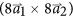with that of the trivial one. When a left-heading wave is excited, it propagates along the edge and can go around the rhombic defect without reflections at the four sharp corners. It also maintains its unidirectional propagation as shown in Fig. 5(a). This result confirms the topological robustness of the edge states against a sharply curved interface.

To conclude, we have designed a 2D acoustic crystal consisting of a triangular array of core-shell cylinders embedded in a water host. We have shown that a topological phase transition can be obtained by using the band inversion mechanism in such a simple system. A pseudo-time-reversal symmetry can be constructed by utilizing the C6ν point group symmetry of the p and d eigenstates at the Γ point, and it follows that pseudo spin-up and spin-down states can be realized in this classical wave system in the same spirit as the quantum spin Hall effect in electronic systems. An effective Hamiltonian is developed for the current acoustic system around the Γ point, and the Hamiltonian unveils the underlying mechanism that links the band inversion to a topological phase transition. Numerical simulations unambiguously demonstrate the unidirectional propagation of the pseudo-spin edge states and the robustness of these edge states against sharp bends. The simple design of our proposed structure suggests that experimental realization is feasible. Since the underlying principle is valid for different scales, we expect that it will have potential applications in manipulating and controlling acoustic waves over a very large frequency range, spanning from infrasound to ultrasound.

## Methods

### Derivation of pseudo-time-reversal symmetry

In the following, we demonstrate that the spatial symmetry of the E1 and E2 representations can be utilized to construct pseudo-time-reversal symmetry23. Let DE1(C6) and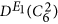denote the E1 irreducible representations of the π/3 and 2π/3 rotations, respectively. Their matrix representations on basis (x, y)T are therefore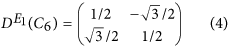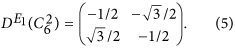It turns out that they can be combined together in a unitary operator, U, as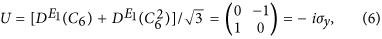where σy is the Pauli matrix. Obviously, U2 = −I. Thus, we can construct an anti-unitary operator, T, as T = UK = −yK, where K is the complex conjugate operator. It follows that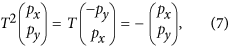which yields T2 = −I. Similarly, the E2 matrix representations of rotational operators C6 and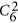on basis (x2 − y2, 2xy)T are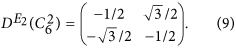And the unitary operator, U, can be constructed as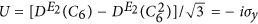. It is easy to check that we also have T2 = −I, the same as in the E1 irreducible representation. Therefore, for both E1 and E2 modes, the pseudo-time-reversal symmetry, T = UK, in the current acoustic system indeed satisfies T2 = −I, which is similar to the real time-reversal symmetry in electronic systems and which also guarantees the appearance of a Kramers doublet at the Γ point. From the derivations shown above, it is clear that the role played by the crystal symmetry of the unit cell in constructing the pseudo-time-reversal symmetry is crucial.

Following this analysis, we can construct the pseudo-spin states as, with p+ (p) being the pseudo spin-up (spin-down) state. On the (p+, p)T basis, the pseudo-time-reversal operator, T′ = UK, [U′ and T′ are defined on the (p+, p)T basis, while U and T are defined on the (px, py)T basis] exhibits the following desired properties: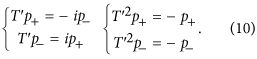From Eq. (10), it is clear that the wave functions (p+, p) are the two pseudo-spin states in the E1 representation of our acoustic system because the pseudo-time-reversal operator, T′, transforms the pseudo spin-up state into a spin-down state, and vice versa. The same conclusion can be made on, which is another pair of pseudo spin-up/spin-down states associated with the E2 representation.

### Numerical simulations

All the numerical simulations presented in this article are performed using COMSOL Multiphysics, a commercial package based on the finite-element method. Figures 1, 2 and 3 are computed using the eigenfrequency study in the pressure acoustics module. The Bloch boundary conditions are imposed on the boundaries of the unit cells. Figures 4 and 5 are calculated using the frequency domain study in the pressure acoustics module, where the acoustic crystal structures are surrounded by perfectly matched layers so that there is no reflected wave from the boundaries of the simulation domains.